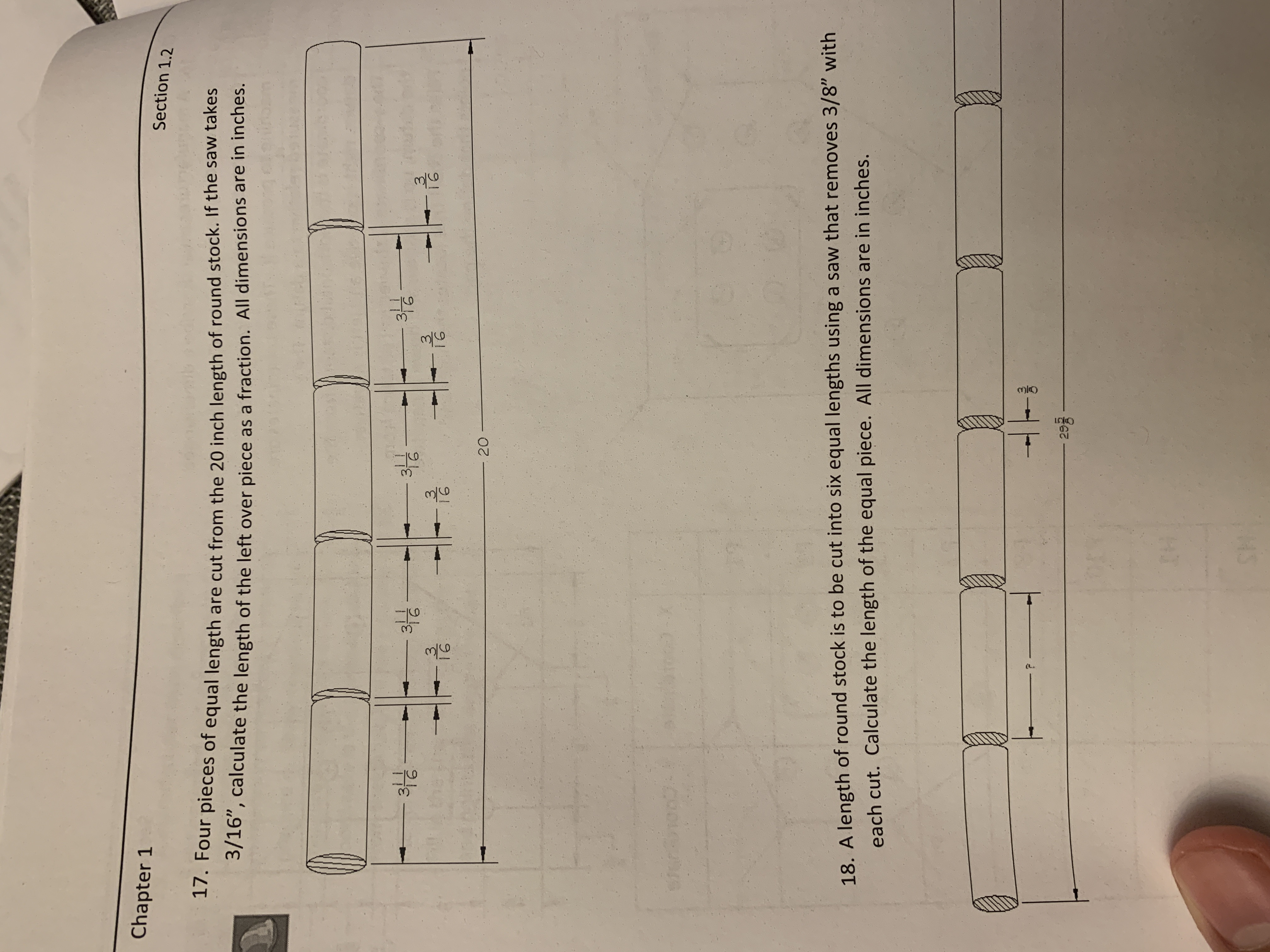# Chapter 1Section 1.2our pieces of equal length are cut from the 20 inch length of round stock. If the saw takes3/16", calculate the length of the left over piece as a fraction. All dimensions are in inches.17. F3İGI 631 620lengths using a saw that removes 3/8" with18. A length of round stock is to be cut into six equaleach cut. Calculate the length of the equal piece. All dimensions are in inches.29g

Question

Number 17.help_outlineImage TranscriptioncloseChapter 1 Section 1.2 our pieces of equal length are cut from the 20 inch length of round stock. If the saw takes 3/16", calculate the length of the left over piece as a fraction. All dimensions are in inches. 17. F 3 İG I 6 3 1 6 20 lengths using a saw that removes 3/8" with 18. A length of round stock is to be cut into six equal each cut. Calculate the length of the equal piece. All dimensions are in inches. 29g fullscreen
check_circleExpert Solution
Step 1

Find the total length of the four cut pieces. Find the total amount of kerf taken with four cuts.

Length of one cut piece = 3-11/16

The amount of kerf taken by the saw for one cut = 3/16

Step 2

The total length of the round stock = 20 inch

The length of the left over piece = 20 ...

### Want to see the full answer?

See Solution

#### Want to see this answer and more?

Solutions are written by subject experts who are available 24/7. Questions are typically answered within 1 hour*

See Solution
*Response times may vary by subject and question
Tagged in

### Math